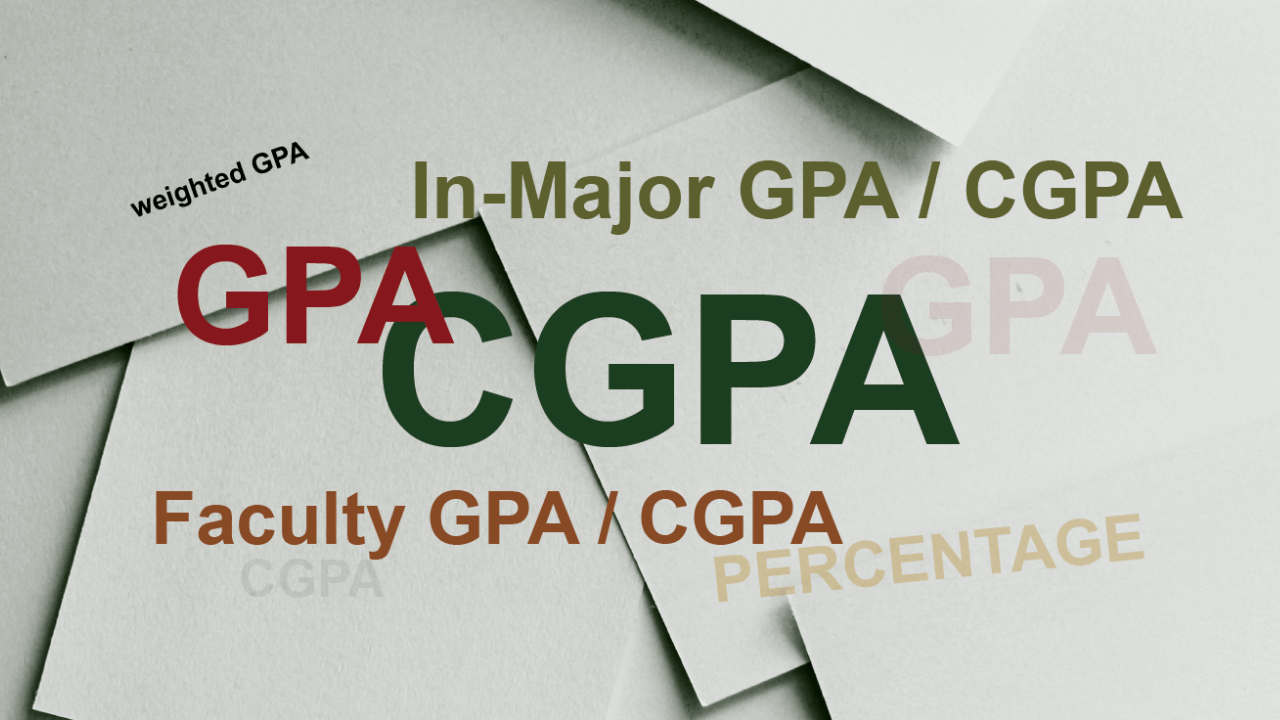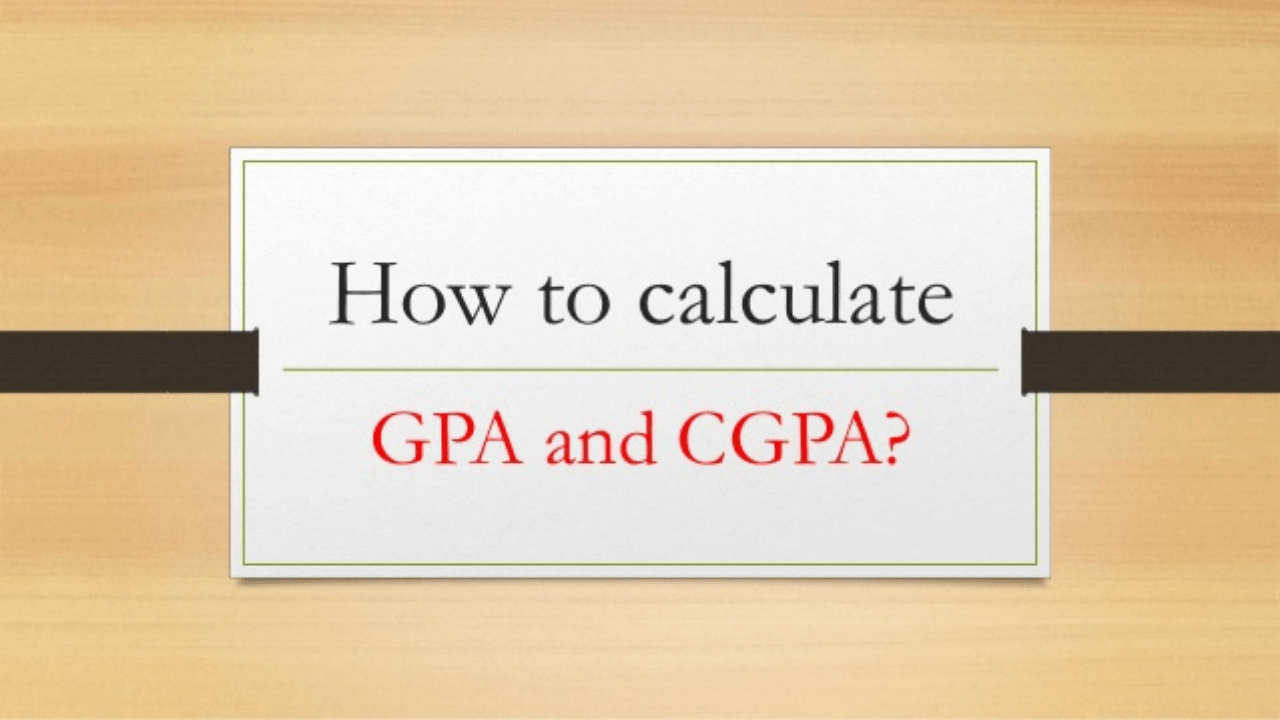# Convert CGPA to Percentage | How to Calculate CGPA from Percentage?

Convert CGPA to Percentage: Every year, the count of students increases appearing for CBSE exams. However, they have much stress in their minds as rewarding marks changes after some years. Thus students need to be very careful in every aspect. However, the system of CGPA calculation confuses them a bit. The feeling is quite different when they think of the final CGPA for their preparation.

However, this system of CGPA put minds to think about calculating the final percentage in every subject. Results are even more important if you are planning to study abroad. International universities demand high previous class scores and standard test results. Most of them also raise the demand for good CGPA. However, the term seems to be quite confusing. But we have tried to resolve it through the blog below. Below you will understand the important aspects while converting CGPA into a percentage.

## How Can You Convert CGPA to Percentage?

According to the Central Board of Secondary Education’s terms and conditions, you need to multiply CGPA by 9.5 to get your percentage. Let us consider an example. Suppose your CGPA is 9.4, then multiply it with 9.5, and the resultant 89.3 is our percentage.

### What does CGPA Actually Define?

CGPA is a Cumulative Grade Point Average. Before you start converting your CGPA into a percentage, it is imperative to define CGPA. Usually, we see CGPA results on our 10th result card. We define CGPA as the average of the collective grade points that we score in the subjects in a year. The grading system is supported by CBSE, which is allotted based on a range of marks. Take an example of grade A. It is allotted to the students obtaining marks between 90-95. Thus their grade point will be 9.

Now, if you have your grade points, how will you calculate CGPA with it? Take the example of a total of 5 subjects. You have grade points of each, and CGPA is the average of all. Sum your grade points and divide by 5. This is how you obtain CGPA. Similarly, if you want to find a percentage in each subject, multiply each grade point by 9.5.### Which is the Right Formula to Convert CGPA to Percentage?

The basic formula of calculating CGPA is through grade points scored in each subject which is further calculated based on a range of marks. Get the grade points of all, add them and divide by a total number of subjects. This is how you calculate your CGPA. Further, to calculate the percentage, multiply your CGPA by 9.5, and the resultant is your percentage.

### Some Important Examples of Calculating CGPA to Percentage

Example 1

Calculate the CGPA if you have 9 in Science, 7 in English, 9 in Hindi, 8 in Social Science, and 10 in Mathematics. Also, calculate the overall percentage and percentage in each subject.

There are a total of 5 subjects for your class as per the question.

Total grade points earned= 9 + 7 + 9 + 8 + 10 = 43.

CGPA= 43/ 5 = 8.6.

Total percentage= 8.6 x 9.5 = 81.7%

Percentage in each subject:

 Name of the Subject CGPA Percentage Science 9 85.5 Maths 10 95 English 7 66.5 Social Science 8 76 Hindi 9 85.5

### CBSE Conversion Certificate

CBSE students are well aware of the marking scheme of the 10th and 12th standards. It is according to the grade points earned in each subject, and the average grade point referred to as CGPA. According to CBSE, grade points are assigned as A1, A2, B1, B2…..

While you are into your exam, you will get a certificate that clearly mentions grade points with the corresponding marks range.

For Example

### What is the Reason for Multiplying 9.5?

Assigning grade systems and CGPA systems is not an everyday task. CBSE that undergone a complete survey for the same. They took a record of students from the past five years and found that the average marks of the students who have earned a band between 91-100 are 95. This is grade A1 with a grade point of 10. Now, after dividing the average marks by 10, we get 9.5. This is how CBSE used the 9.5 factor to calculate the percentage.

### How to Get CGPA from Percentage for Engineering?

Now suppose you are given the percentage of marks obtained. What will be the CGPA earned? To calculate CGPA from percentage, reverse the use of 9.5. Divide 9.5 by percent of marks obtained by you. Suppose your percentage is 94%, then CGPA is 94/ 9.5. The resultant 9.8 is your CGPA.

### CGPA to Percentage in Terms of UPSC

The percentage from CGPA is calculated according to its own terms. One such example is CBSE. However, if you submit your final percent obtained if the university asks for the same, you need to submit according to their guidelines. In case you don’t know the calculation system, UPSC has given an overall formula to calculate the percentage. Suppose your undergraduate level CGPA is 9.0, and your university asks you to submit your percentage, then multiply it with 10 to get your percentage. Your percentage will be 90.

### What are the Benefits of the CGPA system?

• CGPA is basically a grade point system. These grade points help in developing good competition among students. The fact is, none obtains specific marks or ranks in their finals. Thus it helps in the good development of the student’s academic mind.
• Your percentage, according to the CGPA system, will define aggregate marks. CGPA defines your marks in the form of grades.
• It is easy to work with CGPA, while on the other hand, it is good to go with a percentage. Both the systems are important to understand in every aspect.
• Some universities consider both the system. Thus, you need to be sure and clarify your doubts, if any, with CGPA and the percentage system. Various universities provide a formula to calculate percentage from CGPA as per their guidelines. If not so, you can multiply it by 10. This is universally accepted.

### How CGPA Differs from Percentage?

The below table clearly defines how the percentage varies from CGPA:

 CGPA Percentage It is calculated with the average of grade points earned. It is calculated with the average of actual marks obtained. CBSE and other universities use this system Used by various educational institutions Promotes healthy academic mind among students Bring challenges among students. Best performance of the candidates throughout the year. An easy and simple system It is effortless to get a percentage from CGPA. It needs a lot of calculations while calculating the actual percentage.

### CGPA to Percentage in Terms of CBSE

The below table mentions CGPA with the corresponding percentage earned by the students according to CBSE.

 CGPA Percentage 10 95 9.8 93.1 9.6 91.2 9.4 89.3 9.2 87.4 9.0 85.5 8.8 83.6 8.6 81.7 8.4 79.8 8.2 77.9 8.0 76 7.8 74.1 7.6 72.2 7.4 70.3 7.2 68.4 7.0 66.5### How GPA Differs from CGPA?

The grading system is not only limited to CGPA but also about GPA. CGPA is a term used in India, but GPA is recognized in the entire world. GPA is actually the grade points obtained every semester. CGPA is actually the average of all credits earned, marks obtained, and grades obtained. However, if you plan for an abroad study, you need to submit your points according to the universally accepted method. Be prepared to mention your grades in a professional tone. However, if you target any university, you need to understand their calculating policy and mentioned grades accordingly.

### Grade Table According to CBSE

 Marks Grade Grade Points 91-100 A1 10 81-90 A2 9 71-80 B1 8 61-70 B2 7 51-60 C1 6 41-50 C2 5 33-40 D 4 21-32 E1 0-20 E2

### The 10 Point Scale

The 10 point Scale is considered the highest marks obtained by a student. Suppose the highest marks obtained are 94, then 94 will be 100%. If a student gets 85 marks in a particular subject, then CGPA is calculated concerning [100/94] / 85 = 85.10. Hence the CGPA will be 8.5 according to the 10 point scale.

### CGPA to Percentage According to Indian Universities

Universities want to keep records of the percentage of marks obtained. However, the calculation is different according to their guidelines. Hence students need to refer to their official websites for the same. Here are a few examples from the top universities of India.

### CGPA to Percentage According to DU

Delhi University released its Percentage calculation scheme on 11 January 2018. According to the marking scheme:

Percentage = 9.5 x CGPA of all semester

### Convert CGPA To Percentage Mumbai University Calculator

Mumbai University has also recently released its Percentage calculation scheme in 2018. It specifies a different scheme for varied programs. 7 point scale is used in arts, pure science, commerce, and related programs. For engineering, 10 point grade scale is used.

Percentage= 7.25 * CGPA +11

### Convert CGPA To Percentage Anna University

CGPA to % conversion is also declared by Anna University in 2018. However, the scheme keeps changing for many years.

### Convert CGPA To Percentage GTU

Gujarat Technological University also declares its method of calculating % from CGPA.

Percentage: [(CGPA/SPI/CPI-0.5)] *10

### CGPA to Percentage According to APJ Abdul Kalam Technological University

KTU has simplified its way of calculating CGPA. According to them,

CGPA= Sum of (Ci*GPi)/ Sum of (Ci)

### Convert CGPA to Percentage VTU

For students aspiring to get admitted to VTU, the percentage calculation is different from other standards used to date. According to them,

Percentage = [CGPA-0.75] * 10

### FAQ’s on Converting CGPA to Percentage

Question 1.
Define CGPA after learning different aspects.

CGPA is the average obtained by the students out of 10. It is a highly preferred marking scheme for the 10th standard.

Question 2.
How CBSE defines calculating CGPA?

According to CBSE, CGPA is the average of grade points scored in each subject. Suppose we have five subjects in total. Then

CGPA = Total grade points earned / total number of subjects.

Question 3.
What are other additional subjects other than the five main subjects declared on the mark sheet?